Publicité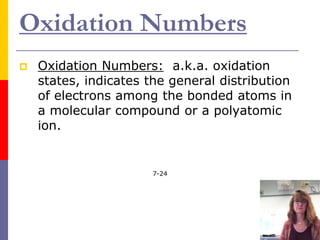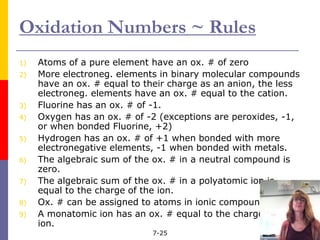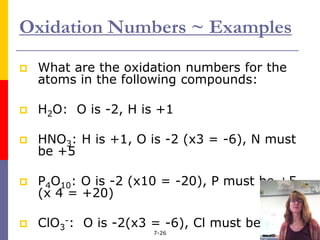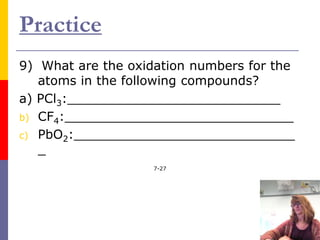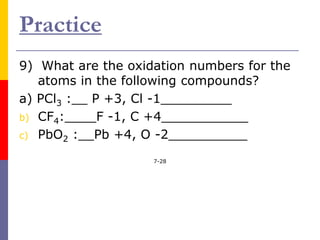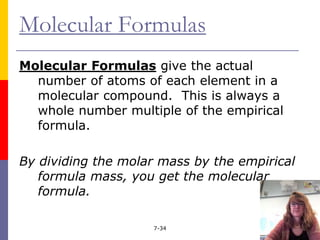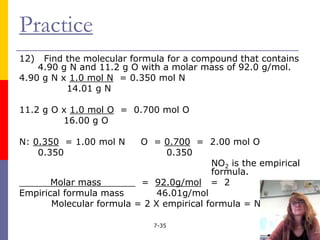1 sur 8
Publicité

### Formulas_and_Naming_Video_4_Optional.pptx

1. Oxidation Numbers  Oxidation Numbers: a.k.a. oxidation states, indicates the general distribution of electrons among the bonded atoms in a molecular compound or a polyatomic ion. 7-24
2. Oxidation Numbers ~ Rules 1) Atoms of a pure element have an ox. # of zero 2) More electroneg. elements in binary molecular compounds have an ox. # equal to their charge as an anion, the less electroneg. elements have an ox. # equal to the cation. 3) Fluorine has an ox. # of -1. 4) Oxygen has an ox. # of -2 (exceptions are peroxides, -1, or when bonded Fluorine, +2) 5) Hydrogen has an ox. # of +1 when bonded with more electronegative elements, -1 when bonded with metals. 6) The algebraic sum of the ox. # in a neutral compound is zero. 7) The algebraic sum of the ox. # in a polyatomic ion is equal to the charge of the ion. 8) Ox. # can be assigned to atoms in ionic compounds. 9) A monatomic ion has an ox. # equal to the charge of the ion. 7-25
3. Oxidation Numbers ~ Examples  What are the oxidation numbers for the atoms in the following compounds:  H2O: O is -2, H is +1  HNO3: H is +1, O is -2 (x3 = -6), N must be +5  P4O10: O is -2 (x10 = -20), P must be +5 (x 4 = +20)  ClO3 -: O is -2(x3 = -6), Cl must be +5 7-26
4. Practice 9) What are the oxidation numbers for the atoms in the following compounds? a) PCl3:___________________________ b) CF4:_____________________________ c) PbO2:____________________________ _ 7-27
5. Practice 9) What are the oxidation numbers for the atoms in the following compounds? a) PCl3 :__ P +3, Cl -1_________ b) CF4:____F -1, C +4___________ c) PbO2 :__Pb +4, O -2__________ 7-28
6. Molecular Formulas Molecular Formulas give the actual number of atoms of each element in a molecular compound. This is always a whole number multiple of the empirical formula. By dividing the molar mass by the empirical formula mass, you get the molecular formula. 7-34
7. Practice 12) Find the molecular formula for a compound that contains 4.90 g N and 11.2 g O with a molar mass of 92.0 g/mol. 4.90 g N x 1.0 mol N = 0.350 mol N 14.01 g N 11.2 g O x 1.0 mol O = 0.700 mol O 16.00 g O N: 0.350 = 1.00 mol N O = 0.700 = 2.00 mol O 0.350 0.350 NO2 is the empirical formula. Molar mass = 92.0g/mol = 2 Empirical formula mass 46.01g/mol Molecular formula = 2 X empirical formula = N2O4 7-35
8. The End!
Publicité